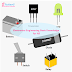Hey, in this article we are going to discuss about the basics of electronics engineering. As an electronics engineering student or teacher or professional, you must have the basic knowledge. So, this article covers - basic electronics concepts, a clear idea of basic electronics topics, and component identification. Before going to read the full article, have you know what is the difference between Electrical and Electronics? Anyway, I am telling in short, electrical mainly deals with power generation, transmission, distribution, and consumption with high voltage and current whereas electronic mostly deals with electricity controlling, and conversion with low voltage and current. Yes, in electronics electricity is used and controlled in a very complicated way. For, example, in a microprocessor, you cannot imagine how electricity is controlled in a complicated way.

## Basic Electronics Topics

Electronics Engineering mainly deals with smaller circuits with more controlling, processing, circuit integration, low power consumption, cheaper and compact designing, portable, higher efficiency, etc. Generally, in the first stage of basic electronics gives you a clear idea of electricity, current, voltage, and power.

### Electricity

Electricity is a form of energy that transfer by the flow of electric current. Electricity or electrical energy is clean energy and is easy to transfer, convert, or store. Nowadays almost 90 percent of our useful work can be done with electrical energy. You can see the examples and applications of electricity in air conditioning, lighting, motor, pumps, elevators, heater, cooler, fan, etc.

### Current

Current is the flow of electrons through a conductive path, object, or any material. The flow of electric current depends upon the pressure or potential difference or voltage between two ends of the conductor and the internal resistance of the conductor. The unit of current is ampere.

### Voltage

In simple words, voltage is the electric pressure that forces to flow the electrons through a conductor. And this pressure is created when a potential difference is applied across the two ends of that conductor. The unit of voltage is volt.

### Power

Mathematically, power is the multiplication of voltage and current. Power is measured by the flow of current from a power source to load with applied voltage. The unit of power is the watt.

## Learning about Basic Electronic Component

Learning about electronic components will strengthen your basic knowledge in electronics engineering.

### Resistor

A resistor is a passive component that opposes the flow of current in an electrical or electronic circuit. Another function of a resistor or resistive material is to convert electrical energy into heat energy. The property of a resistor is called resistance. The unit of resistance is the ohm.

### Inductor

An inductor is also a passive component. The main function of an inductor is to oppose the changes in the flow of current through it. The inductor blocks the alternating current or AC and allows the Direct Current or DC. The property of the inductor is called inductance. The unit of inductance is henry.

### Capacitor

A capacitor is a passive component whose main function is to store electrical energy in the form of an electric charge. The property of a capacitor is opposite to an inductor means, the capacitor blocks the direct current(DC) and allows the alternating current(AC). The property of a capacitor is called Capacitance. And the unit of the capacitor is called farad. Basically, the capacitor is a two-terminal device.

### Semiconductor Devices

Having a background in electronics engineering, you must know about important semiconductor devices such as transistors, diodes, and ICs. IC means Integrated Circuit. In our blog, we have so many articles about those semiconductor devices. So, you can read about them.

## Basics symbols in Electronics Engineering

You must know about basic electronic symbols such as a resistor, inductor, capacitor, transistor, and other electronic components. Not only electronic components, but even having knowledge of circuit symbols, electronic drawing symbols are also very important.

Here, I give some links to important articles related to the electronics engineering. So, you can read these articles to increase your basic knowledge.

Electronics Engineering Basic Knowledge for AllReviewed by Author on 6:13 pm Rating: 5